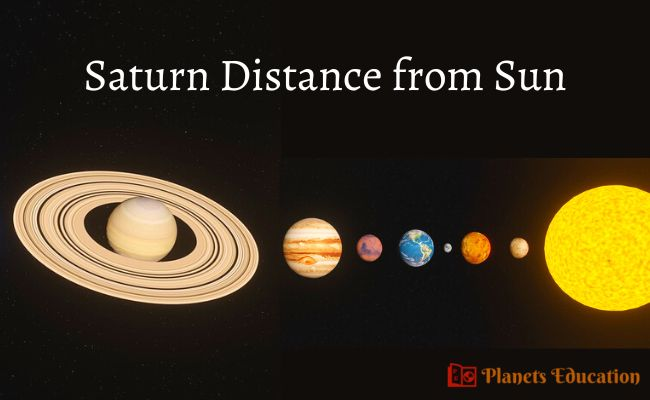# Saturn Distance from Sun – How Far is Planet Saturn?The planet Saturn is the sixth planet from the Sun and it is the second-largest planet after Jupiter. Here you will know, Saturn distance from the Sun in many units, like Kilometers, Miles, AU, and Light-Years.

Saturn is the most distant planet that we can see easily with the naked eye in the night sky. But you may have questions about “how far is Saturn from the Sun” and “how far away is Saturn from the Earth”?

The average distance between Saturn Planet and the Sun is around 9.58 AU. The orbital motion of planet Saturn around the Sun is not ideal in a circular motion. It has an elliptical orbit around the sun. So, the distance between Sun and Saturn always varies.

## How far is Saturn from the Sun

In our solar system, all planets orbit around the Sun in an elliptical way. Due to their elliptical orbit, the distance between these planets and the sun always varies. The closest distance of any world from the sun is called perihelion and the farthest distance between a planet and the sun is called aphelion.

Some planets are nearer to Sun, like Planet Mercury, and Venus. But some planets are quite far from the Sun, like Planet Saturn, Uranus, and Neptune

This article explains the minimum, maximum, and average Saturn distance from Sun in different units.

Below is mentioned Saturn to Sun distance in kilometers:

• The average distance between Sun and Saturn is 1,433.52 million km.
• The closest distance of Saturn from the Sun is 1,352.55 million km.
• And the farthest Saturn and Sun distance is 1,514.50 million km.

These distances between Saturn and Sun were in kilometer units. Below you will find other units.

### Saturn to Sun distance in miles

• Average distance – 890.75 million miles.
• The closest distance – is 840.43 million miles.
• The farthest distance – is 941.06 million miles.

### Distance between Saturn and Sun in AU

• Average distance – 9.58 AU.
• The closest distance – is 9.04 AU.
• The farthest distance – is 10.12 AU.

### Saturn to Sun distance in light-years

• Average distance – 0.00015 light-years.
• The closest distance – is 0.00014 light-years.
• Farthest distance – 0.00016 light-years.

These were distances between the planet Saturn and Sun in different units (kilometers, miles, AU, and light-years).

Also Check:-